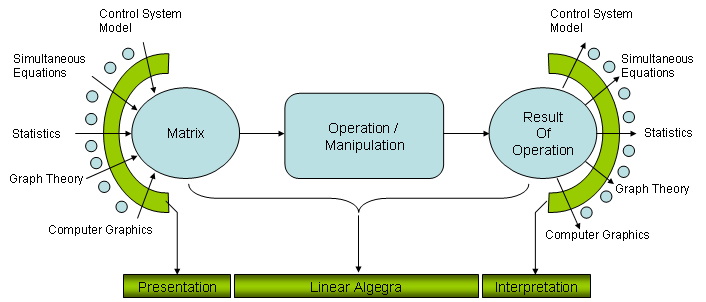Engineering Math - Matrix Overview   Matrix would be one of the most important and widely used mathematical tool in engineering. I personally think that you would adapt to almost any engineering field if you are familiar with Differential Equation and Matrix. Personally Matrix is one of the intriguing concept and tools to me. I know many of you would not agree with me especially if you are already got sick and tired of so many calculations and struggling with Linear Algebra course.     How we use Matrix to solve a real life problem   My understanding of use model for Matrix in engineering field is as shown in the following illustration. Try to interpret the illustration from left to right.i) First, represent your problem in real life into a Matrix form (You will see a couple of examples of these represetations from following sections). ii) And then you would apply various mathematical operations to the Matrix and get the outcome of the operation. (Determining what kind of operatioin you should perform and understand why you should apply those operations should be the critical part at this step). iii) Finally you have to interpret the result of step ii) into your real life situation.   I think one of the biggest reason why your linear algebra course does not interest you (even scarying you away) is that it focus mostly in step ii) and in many case you would not feel such a strong interest to anything which does not show any close relationships to real life problems.   In this page, I would provide you some examples to trying to show you the step i) and step iii) as much as possible and I will keep adding those examples. Of course,  only a couple of examples explained here would not be enough to give you strong motivation and understandings on Matrix. But I hope it can at least be a small trigger.     Presentation to Matrix   Before I put my own words, I would like to strongly recommend you to see an excellent lecture by Proffer Stephen Boyd. Introduction to Linear Dynamical System. If you want to see the full course, visit here.  Interestingly, this lecture shows exactly the approach that I want to take when I am studying Matrix.     Mathematical Operation and Properties of Matrix   There are so many different kind of mathematical operator, special form of matrix and various indicator showing special properties of a matrix. You may be familiar with some of the operators and concept from High school math (e.g, Inner Product) and there are some of the concept you might have heard so many times from Linear Algebra class (like Eigenvector, Eigenvalue, Determinent etc), and there are a lot of things you might have heard from various source but doesn't have clear understanding. It is no point of trying to memorize all of these concept, just look into the definition and trying to think of practical meaning everytime you see them used.